Linear differential operator

This article defines a property that can be evaluated for a differential operator on a differential manifold (viz a linear map from the space of differentiable functions to itself)

Definition

Given data

A connected differential manifold$M$. The$\R$-algebra of$C^\infty$-functions from$M$ to$\mathbb{R}$ is denoted by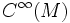$C^\infty(M)$.

Definition part

A linear differential operator is a map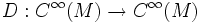$D: C^\infty(M) \to C^\infty(M)$ which has order$k$ for some integer$k$, where an operator$D$ is said to be of order$k$ if$D$ can be written as a fintie linear combination of compositions of derivations (vector field operators) with each composition involving at most$k$ derivations.

Equivalently,$D$ is of order$k$, if for any functions$f_1, f_2, \ldots, f_k$: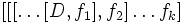$[[[\ldots[D,f_1],f_2]\ldots f_k]$

is an ordinary scalar function, where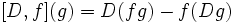$[D,f](g) = D(fg) - f(Dg)$

Particular cases

It turns out that first-order linear differential operators can be expressed in the form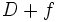$D + f$ where$D$ is a derivation and$f$ is a function (Acting by pointwise multiplication).